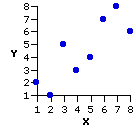Subchapter 3b.
Rank-Order Correlation

The version of correlation examined in the main body of Chapter 3 applies to those cases where the values of X and of Y are both measured on an equal- interval scale. It is also possible to apply the apparatus of linear correlation to cases where X and Y are measured on a merely ordinal scale. When applied to ordinal data, the measure of correlation is spoken of as the Spearman rank- order correlation coefficient, typically symbolized as rs.

Suppose, for example, that two experts, X and Y, were asked to rank N=8 items with respect to some dimension germane to their field of expertise (rank#1=highest, rank#8=lowest). To make it specific, you can imagine two physicians ranking 8 patients with respect to the severity of their disease; two psychotherapists ranking 8 patients with respect to the likelihood of improvement; two wine experts ranking 8 wines from best to worst; two statisticians ranking 8 statistical concepts with respect to their fundamental importance; or whatever else it might be that strikes your fancy.

As a token of my liberal-mindedness—for I am one of those benighted souls who find all wines to taste suspiciously like vinegar—I will use the image of the wine experts. The following table shows the rankings from 1 to 8, best to worst, of two experts, X and Y.

 wine X Y a b c d e f g h 12345678 21534786As you can see from the accompanying graph, there is a substantial degree of agreement between the rankings of the two experts. Plug the bivariate values of X and Y into the formulaic structure given in the main body of Chapter 3,
 r = SCXY sqrt[SSX x SSY] and you will find   r = +.83   r2 = .69.

As it happens, these are exactly the same values you will get when you calculate the Spearman coefficient, rs. The simple reason for this is that r and rs are algebraically equivalent in the case where the values of X and Y consist of two sets of N rankings. The only advantage of rs is that the calculations are easier if you are doing them by hand. [Note, however, that rs is precisely equal to r only when the rankings within X and Y are the consecutive integer values: 1, 2, 3, and so on, with no ties. With tied ranks there will tend to be discrepancies between rs and r. If the proportion of tied ranks is fairly large, you would be better advised to plug your rankings for X and Y into the standard formula for r.]

The Simple Formula for rs, for Rankings without Ties

 wine X Y D D2 a b c d e f g h 12345678 21534786 —11—211—1—12 11411114 N = 8-∑D2 = 14
Here is the same table you saw above, except now we also take the difference between each pair of ranks (D=XY), and then the square of each difference. All that is required for the calculation of the Spearman coefficient are the values of N and-D2, according to the formula

 rs = 1 — 6∑D2N(N2—1)

If this formula seems a bit odd to you, you are in good company. Generations of statistics students have been presented with it, and generations have puzzled over such mind- bending questions as: why do you start out with "1" and subtract something from it?; where does that N(N21) in the denominator come from?; and, above all, how does that peculiar "6" get into the numerator?

Here are the answers to these age-old questions in a nutshell.

• For any set of N paired bivariate ranks, the minimum possible value of-D2 occurs in the case of perfect positive correlation. In this case, rank 1 for X is paired with rank 1 for Y, rank 2 for X with rank 2 for Y, and so on. Each value of D will accordingly be equal to zero, and so too will be the sum of the squared values of D.

• Conversely, the maximum possible value of-D2 occurs in the case of perfect negative correlation. This maximum possible value is in every instance equal to
 maximum-∑D2 = N(N2—1)3

Thus, for N=8 with perfect negative correlation:T
 item X Y D D2 -∑D2 = 168   8(82—1)/3 = 168 a b c d e f g h 12345678 87654321 —7—5—3—11357 492591192549

• The ratio of the observed-D2 to its maximum possible value will therefore be equal to zero in the case of perfect positive correlation, to +1.0 in the case of perfect negative correlation, and to +.50 in the case of zero correlation.

 -∑D2N(N2—1)/3 = 3∑D2N(N2—1)

Double this ratio, subtract it from 1, and voila! you have a quantity that will be equal to +1.0 in the case of perfect positive correlation, to 1.0 in the case of perfect negative correlation, and to zero in the case of zero correlation.

 rs = 1 — 6∑D2N(N2—1)

And here, finally, is the calculation of rs for the example with which we began:

 wine X Y D D2 a b c d e f g h 12345678 21534786 —11—211—1—12 11411114 N = 8-∑D2 = 14

 rs = 1 — 6∑D2N(N2—1) = 1 — 6 x 148(82—1) = +.83 r2s = .69

The meanings of rs and r2s in a rank- order correlation are essentially the same as those of r and r2 in a correlation based on equal- interval data. For the present example, r2s=.69 means that the covariance between the X and Y rankings is 69% as strong as it possibly could be, and the positive sign of rs=+.83 signals that this covariation occurs along the upward slant, with higher values of X tending to be associated with higher values of Y, and vice versa. However, I would not recommend taking the parallels much farther than this. In particular, I think it would not make much sense to subject bivariate rankings to the predictive apparatus of linear regression.

End of Subchapter 3b.